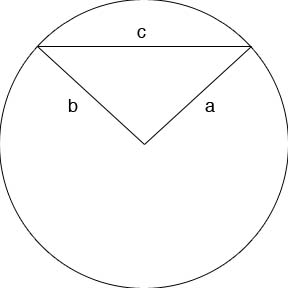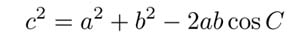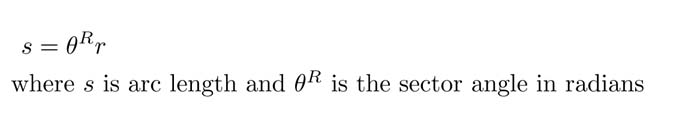SEARCH HOMEMath Central Quandaries & QueriesBenson: If chord length, radius are given, How to find the sector angle and arc-lengthHi Benson,

Since the triangle that is created by the radii and the chord do not make a right triangle, we have to use the Law of Cosine. In my diagram, the chord length is c and the radii are a & b.Using the law of cosinesolve for angle C which is the angle across from side c, this is your sector angle. You can then use the arc length formulaHope this helps,

JaniceMath Central is supported by the University of Regina and The Pacific Institute for the Mathematical Sciences.